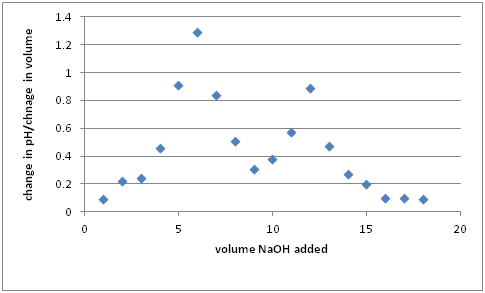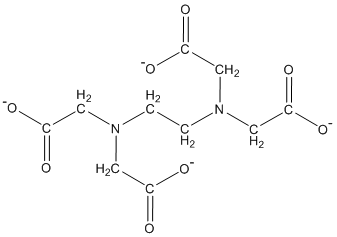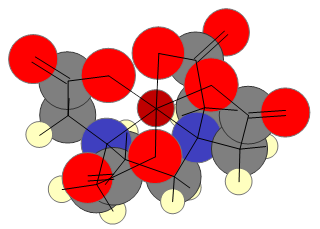# Titrimetry, Alkalinity, and Water Hardness

$$\newcommand{\vecs}{\overset { \rightharpoonup} {\mathbf{#1}} }$$ $$\newcommand{\vecd}{\overset{-\!-\!\rightharpoonup}{\vphantom{a}\smash {#1}}}$$$$\newcommand{\id}{\mathrm{id}}$$ $$\newcommand{\Span}{\mathrm{span}}$$ $$\newcommand{\kernel}{\mathrm{null}\,}$$ $$\newcommand{\range}{\mathrm{range}\,}$$ $$\newcommand{\RealPart}{\mathrm{Re}}$$ $$\newcommand{\ImaginaryPart}{\mathrm{Im}}$$ $$\newcommand{\Argument}{\mathrm{Arg}}$$ $$\newcommand{\norm}{\| #1 \|}$$ $$\newcommand{\inner}{\langle #1, #2 \rangle}$$ $$\newcommand{\Span}{\mathrm{span}}$$ $$\newcommand{\id}{\mathrm{id}}$$ $$\newcommand{\Span}{\mathrm{span}}$$ $$\newcommand{\kernel}{\mathrm{null}\,}$$ $$\newcommand{\range}{\mathrm{range}\,}$$ $$\newcommand{\RealPart}{\mathrm{Re}}$$ $$\newcommand{\ImaginaryPart}{\mathrm{Im}}$$ $$\newcommand{\Argument}{\mathrm{Arg}}$$ $$\newcommand{\norm}{\| #1 \|}$$ $$\newcommand{\inner}{\langle #1, #2 \rangle}$$ $$\newcommand{\Span}{\mathrm{span}}$$

## Titrimetry

Purpose: This module provides an introduction to Titrimetry. In this module the basic theory and applications of titrimetry in water quality analysis will be presented at a level that assumes a basic general chemistry background.

Learning Objectives: At the end of this content, students will be able to:

1. Identify the key components of general titrimetric methods.
2. Evaluate the feasibility for using a titrimetric method for a specific sample.

Titrimetry is often used in determining the concentration of acids or bases in solutions, but can also be used in determining the concentration of other analytes. In any titration, you add a titrant to a titrand. You need to know the chemical reaction that is occurring so that you know the stoichiometry between the titrant and the titrand.

Q1. In an acid-base titration, what is the chemical reaction that is occurring and how would you determine the endpoint, when all of the unknown had reacted with the titrant?

Q2. What might be the difference between using an acid-base indicator and a pH electrode in measuring the endpoint for an acid-base titration?

If you think about this scenario, you have basically identified that there is some way to discriminate when a stoichiometrically equivalent amount of two different solutions are mixed.

In a broad sense, titrimetric methods require two solutions to be combined while accurately measuring at least one of the volumes, and often both volumes. The method also requires some way to determine an endpoint to be able to use stoichiometry to calculate the amount of the unknown analyte in the sample. In order for the titrimetric method to be viable, the chemical reaction must be complete and fast.

Since you are using a chemical reaction to compare the amounts of two chemicals, the minimum concentration that can be detected usually is rather high (normally over 100 ppm). For example, it is difficult to use any acid or base solution with a concentration below 0.001 M, primarily due to impacts of water ionization or the presence of dissolved CO2, which produces some carbonic acid in the solution.

## Alkalinity or Acid Neutralizing Capacity (ANC)

Purpose: The purpose of this assignment is to introduce concepts of titrimetry as they pertain to the determination of ANC and water hardness of water samples.

Learning Objectives: At the end of this assignment, students will be able to:

1. Understand the chemistry occurring in the analysis of ANC.
2. Be able to determine ANC in a water sample.

Acid Neutralizing Capacity is the capacity of a water sample to neutralize added acid (the standard method can be found in the EPA National Field Manual for the Collection of Water Quality Data, chapter 6.6, at http://water.usgs.gov/owq/FieldManual/). ANC is measured on a water sample as collected, while alkalinity is measured on filtered (0.45 μm) samples. Alkalinity is often measured to check the charge balance of a solution or to identify how susceptible a water system is to acidification from inputs such as acid rain.

Q1. How is ANC different than the pH of a solution?

Q2. What are some likely chemical species present in natural water that would neutralize an added acid?

Q3. How could particles filtered out of a water sample neutralize an acid?

Q4. If pKa1 of carbonic acid is 6.352 and pKa2 is 10.329, what carbonate species would primarily be present at pH 7.0? As you adjust the pH to around 4.5, how would the dominant species change?

The primary method to determine ANC is by titrating the water sample with sulfuric acid to neutralize the bicarbonate species.

Q5. For most surface water samples, the method calls for a sample volume of either 50 mL or 100 mL—How would you accurately measure this volume?

Q6. The analysis requires a known concentration of H2SO4, usually 0.1600N or lower. How would you prepare and standardize this titrant

During the titration, the solution is stirred and the pH is measured after each addition of titrant. The endpoint of the titration is the point at which all of the bicarbonate species has been neutralized. Therefore, as you titrate the solution, care must be taken to titrate slowly to a pH of 4.0 or lower, to obtain sufficient data to define the endpoint.

Q7. Sketch an approximate plot of how the pH of a solution of calcium carbonate (a representative weak base) would change as it is titrated with a strong acid. Where is the endpoint of the titration on this plot?

To analyze the ANC data, you would create an analogous plot of the pH versus of the volume of titrant, and calculate the change in pH per change in volume for each data point. The greatest change in pH per change in volume will yield the equivalence points for the titration. (If you start above pH 9, you should have two places of really high change in pH per change in volume)

A plot of representative data can be seen below, where the greatest change in pH per volume can be seen at 6 mL and 12 mL additions.A

## Water Hardness

Analytes other than acids and bases can also be measured via titrimetry. One such use of titrimetry is the determination of water hardness (EPA method 130.2-- http://www.epa.gov/region6/6lab/methods/130_2.pdf). Hard water (water containing a high concentration of multivalent ions such as Ca2+ and Mg2+) has a tendency to form an insoluble scale on the inside of pipes. Hard water can also form a sparingly soluble precipitate with soap (“soap scum”). This precipitate makes it hard to get suds to form and good detergent action in water high in Ca2+ and Mg2+, hence the name “hard water”. A complexing molecule such as ethylenediaminetetraacetic acid (EDTA) is commonly used to determine the concentration of calcium and magnesium in natural water samples.

Theory

The chemical structure for the fully deprotonated form of EDTA is shown below:There are six binding sites on the EDTA molecule. Four of these sites are the carboxylic acid groups and two of these sites are the nonbonding electron pairs on the nitrogen atoms. All six of these sites are used to interact electrostatically with multivalent cations to form a coordination compound. In this case, EDTA serves as the electron donor group or ligand.

Q1. How can you ensure that the four carboxylic acid sites are deprotonated to ensure the ability to bind with Ca2+ and Mg2+?

Ligands with two or more electron donor groups belong to a class of compounds called chelates. The word chelate is derived from the Greek word for claw. Because EDTA has six electron donor groups, it is referred to as a hexadentate chelating agent. The EDTA molecule can wrap around a multivalent cation such that the electron donor groups are positioned around the central metal ion to form an octahedral structure. Below is a three-dimensional representation of an EDTA molecule bound to a ferric ion.The presence of so many electron donor groups on the EDTA molecule provides it with some beneficial properties with respect to complexometric titrations.

1. EDTA forms a 1:1 complex with metal ions, so the titration endpoint is well defined.
2. Metal-EDTA complexes are highly stable so the reaction lies almost completely on the product side (Kf >>1), a necessary requirement for titration work.

Q2. What metals would EDTA likely form stable complexes with in most water samples? How selective is this process?

Q3. The endpoint of a titration is determined using an indicator. What would be the general features of an indicator that could be used to determine the endpoint of a water hardness titration?

There is a class of indicators that change color as a metal binds to the indicator. For EPA method 130.2 Eriochrome Black T (EBT) is used to determine total water hardness. In the case of EBT, the indicator is wine-red in the presence of metal ions and blue when the indicator does not have a metal bound to it.

Q4. When the EBT is added to your sample, what color would you expect for the indicator?

This method is used to determine total water hardness, which is the combined concentration of Ca2+ and Mg2+. With a slight modification it can also be used to determine the individual concentrations of Ca2+ and Mg2+ in solution. The modification involves adjusting the pH to a higher or more basic value. Since the pH is a higher value, a different indicator, hydroxynapthol blue (HB) is used. In the case of HB, the indicator is raspberry-red in the presence of metal ions and lavender when no metal ions are complexed. Let us consider how this modification works to allow the analysis of an individual analyte.

Q5.  How might raising the pH potentially cause metals to precipitate?

The chart below lists the solubility product constants of the hydroxide complexes of several common cations in water.

Q6. Which metal would precipitate last as its hydroxide complex? This is the metal then available for titration using the HB indicator after you adjusted the solution pH to 12.0.

Cation

Equilibrium

Ksp

Al3+

Al(OH)3 (s) ↔ Al3+ (aq) + 3 OH- (aq)

4.6 x 10-33

Ca2+

Ca(OH)2 (s) ↔ Ca2+ (aq) + 2 OH- (aq)

6.5 x 10-6

Fe2+

Fe(OH)2 (s) ↔ Fe2+ (aq) + 2 OH- (aq)

8 x 10-16

Fe3+

Fe(OH)3 (s) ↔ Fe3+ (aq) + 3 OH- (aq)

1.6 x 10-39

Mg2+

Mg(OH)2 (s) ↔ Mg2+ (aq) + 2 OH- (aq)

7.1 x 10-12

Q7. If you have measured the total water hardness using EBT as the endpoint and the concentration of the Ca2+ using HB as the endpoint, how could you determine the concentration of Mg2+

Q8. Have you made any assumptions in performing this calculation?

Q9. The concentration of the titrant needs to be known when performing a titration. This concentration is determined by standardizing the titrant. How could you standardize the EDTA solution?

An arbitrary scale has been set up to define the “hardness” of water (the ppm concentrations represent the combined concentration of Ca2+ and Mg2+).

“Soft” = 0-60 ppm

“Moderately hard” = 61-120 ppm

“Hard” = 121-180 ppm

“Very hard” = over 180 ppm

Titrimetry, Alkalinity, and Water Hardness is shared under a CC BY-NC-SA license and was authored, remixed, and/or curated by LibreTexts.